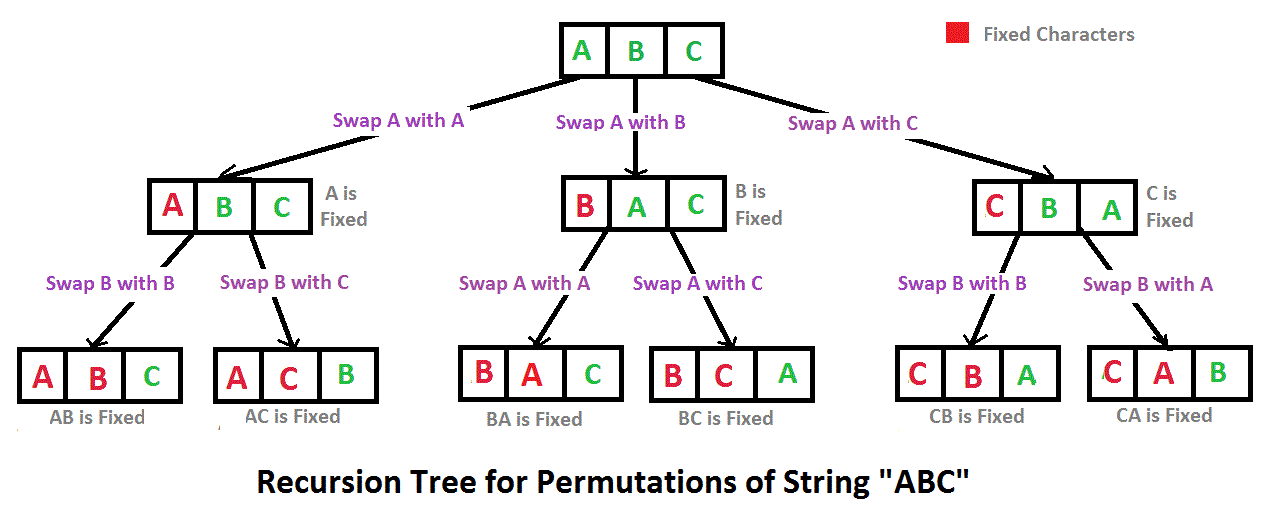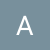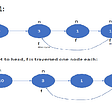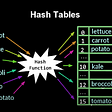# Algorithm:

`class Solution {    Map<TreeNode, TreeNode> parent;    public List<Integer> distanceK(TreeNode root, TreeNode target, int k) {        parent = new HashMap();        dfs(root, null);        Queue<TreeNode> queue = new LinkedList();        Set<TreeNode> seen = new HashSet();        queue.add(target);        int level=0;        seen.add(target);        while(!queue.isEmpty()){            int size= queue.size();            if(k==level) break;            while(size>0){                TreeNode curr =queue.poll();                if(curr.left!=null&&!seen.contains(curr.left)){                    queue.add(curr.left);                    seen.add(curr.left);                }                if(curr.right!=null&&!seen.contains(curr.right)){                    queue.add(curr.right);                    seen.add(curr.right);                }                if(parent.get(curr)!=null&&!seen.contains(parent.get(curr))){                    queue.add(parent.get(curr));                    seen.add(parent.get(curr));                }                size--;            }            level++;        }//now we have elements in queue that are k distance apart from target        int n= queue.size();        List<Integer> list = new ArrayList();        for(int i=0;i<n;i++){            list.add(queue.poll().val);        }        return list;    }    public void dfs(TreeNode node, TreeNode par) {        if (node != null) {            parent.put(node, par);            dfs(node.left, node);            dfs(node.right, node);        }    }}`

--

--

--

## More from Abhinay Gupta

Love podcasts or audiobooks? Learn on the go with our new app.

## String Permutations in JS## Code a Taxi app with Ionic, NodeJS and MySQL — Part 2## An Update on ES6 Modules in Node.js## Sync with Multiple v-models in Vue 3 using Composition API## Storybook 6.3## 5 Ways to Simplify Your React Hooks## Greedy Algorithm## Cycle detection in a linked list## Introduction: What is a Hash Table## A* Search Algorithm